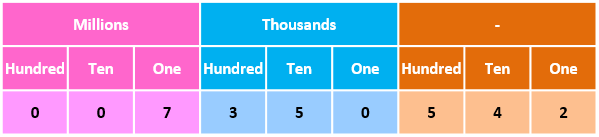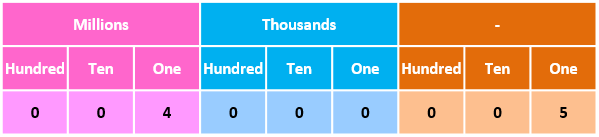## Words to Figures (Words to Numerals)

Practice Unlimited Questions

#### 1. Understanding the need for writing numbers in figures or numerals

Numbers that are expressed in figures or numerals are easy to write, easy to use, easy to remember, and easy to work with.

It's the most common and natural way of expressing a number.

#### 2. Express seven million, three hundred fifty thousand, five hundred forty two in figures.

Step 1: Mark the keywords "million" and "thousand" and then split up the number into groups of 3 like this:
• Seven million --> 007 million (write "7" as three bits)
• Three hundred and fifty thousand --> 350 thousand (write "350" as three bits)
• Five hundred and forty two --> 542 (write "542" as three bits)
Step 2: Each of the three groups above has 3 digits. Put them in the table below like this.Step 3: Drop the initial zeroes in the number in the above table and write seven million, three hundred fifty thousand, five hundred and forty two in figures as 7 350 542.

#### 3. What is four million and five in numerals?

Step 1: Mark the keywords "million" and "thousand" and then split up the number into groups of 3 like this:
• Four million --> 004 million (write "4" as three bits)
• Zero thousand --> 000 thousand (since there is nothing for thousand, we put a 0 for each of the three bits)
• Five --> 005 (write "5" as three bits)
Step 2: Each of the three groups above has 3 digits. Put them in the table below like this.In numerals, four million and five as 4 000 005.